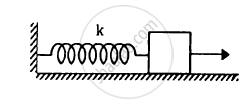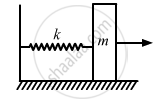Advertisement Remove all ads

# The Spring Shown in Figure is Unstretched When a Man Starts Pulling on the Cord. the Mass of the Block is M. If the Man Exerts a Constant Force - Physics

Sum

The spring shown in figure is unstretched when a man starts pulling on the cord. The mass of the block is M. If the man exerts a constant force F, find (a) the amplitude and the time period of the motion of the block, (b) the energy stored in the spring when the block passes through the equilibrium position and (c) the kinetic energy of the block at this position.Advertisement Remove all ads

#### Solution(a) We know-
f = kx

$\Rightarrow x = \frac{F}{k}$

$\text { Acceleration }= \frac{F}{m}$

Using the relation of time period of S.H.M.,

$\text { Time period }, T = 2\pi\sqrt{\frac{\text { Displacement } }{\text { Acceleration }}}$

$= 2\pi\sqrt{\frac{\left( \frac{F}{k} \right)}{\left( \frac{F}{m} \right)}} = 2\pi\sqrt{\frac{m}{k}}$

Amplitude = Maximum displacement $= \frac{F}{k}$

When the block passes through the equilibrium position, the energy contained by the spring is given by,

$E = \frac{1}{2}k x^2 = \frac{1}{2}k \left( \frac{F}{k} \right)^2 = \frac{1}{2}\left( \frac{F^2}{k} \right)$

(b) At the mean position, potential energy is zero.
Kinetic energy is given by,

$\frac{1}{2}k x^2 = \frac{1}{2}\frac{F^2}{k}$

Is there an error in this question or solution?
Advertisement Remove all ads

#### APPEARS IN

HC Verma Class 11, 12 Concepts of Physics 1
Chapter 12 Simple Harmonics Motion
Q 18 | Page 253
Advertisement Remove all ads
Advertisement Remove all ads
Share
Notifications

View all notifications

Forgot password?